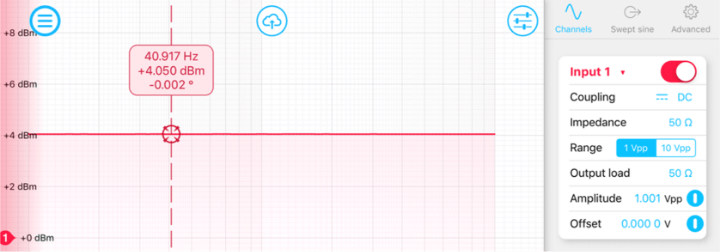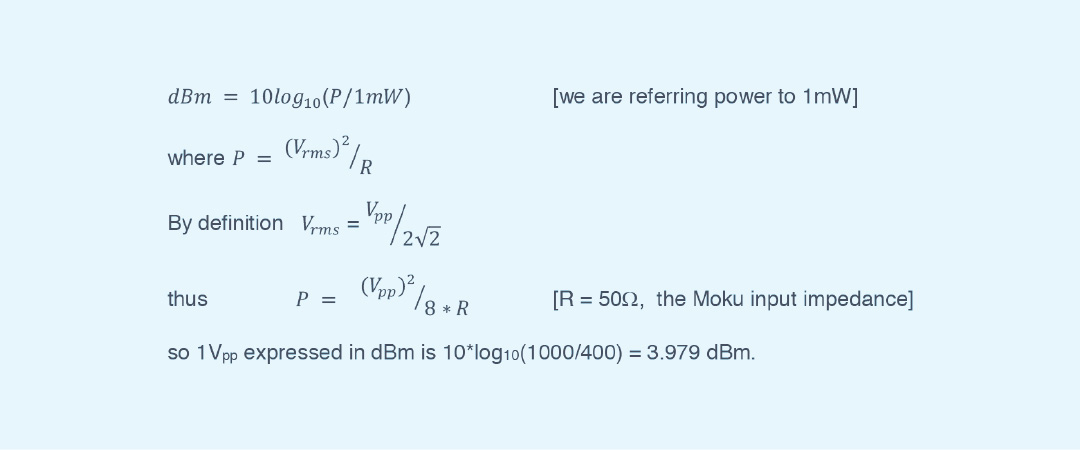##Frequency Response Analyzer

### Overview

Moku:Lab’s Frequency Response Analyzer can be used to measure a system’s frequency response from 10 mHz up to 120 MHz.

### Docs

Specs
Moku:Lab Spec Sheet

### FAQ

How can I plot the ratio of Input 1 and Input 2?

By default, each channel shows the ratio of the input to the output, In / Out. This is useful for measuring the transfer function of a device under test. The math channel allows you to plot different combinations of Ch 1 and Ch 2. If the output amplitudes of both channels are set to the same value, then viewing the math channel as Ch 1 / Ch 2 will show the ratio In 1 / In 2, since the outputs are the same.

How is this magnitude in dBm calculated in Moku:Lab’s FRA?

The Frequency Response Analyzer (FRA) provides response plots of magnitude and phase. While the phase is expressed in degrees, the magnitude is expressed in terms of dBm power. This is a log scale of power expressed in dB relative to 1 milliWatt.

How is this magnitude in dBm calculated in Moku:Lab’s FRA?

Let us take a simple example. We will set the swept sine wave output of the FRA to 1Vpp, configure 50 Ω input and output impedance and for this example, a sweep from 10Hz to 1kHz. Then with a coax cable connecting output 1 of the Moku:Lab to input 1, we run a sweep of the FRA. You should see a flat amplitude response at 4.0 dBm.How is the 4.0 dBm is calculated?

It comes from this formula (valid for sinusoidal signals):